## Important Questions for Class 10 Maths Chapter 5 Arithmetic Progressions

### Arithmetic Progressions Class 10 Important Questions Very Short Answer (1 Mark)

Ap Questions Class 10 Question 1.
Find the common difference of the AP $$\frac{1}{p}, \frac{1-p}{p}, \frac{1-2 p}{p}, \dots$$. (2013D)
Solution:
The common difference,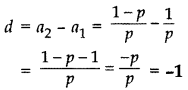Arithmetic Progression Class 10 Questions Question 2.
Find the common difference of the A.P. $$\frac{1}{2 b}, \frac{1-6 b}{2 b}, \frac{1-12 b}{2 b}, \ldots$$. (2013D)
Solution:
The common difference, d = a2 – a1 = $$\frac{1-6 b}{2 b}-\frac{1}{2 b}$$
= $$\frac{1-6 b-1}{2 b}=\frac{-6 b}{2 b}$$ = -3

Important Questions Arithmetic Progression Class 10 With Solutions Pdf Question 3.
Find the common difference of the A.P. $$\frac{1}{3 q}, \frac{1-6 q}{3 q}, \frac{1-12 q}{3 q}, \dots$$. (2013D)
Solution:
Common difference, d = a2 – a1 = $$\frac{1-6 q}{3 q}-\frac{1}{3 q}$$
= $$\frac{1-6 q-1}{3 q}=\frac{-6 q}{3 q}$$ = -2

For more detail and in depth learning regarding our common difference calculator, find arithmetic sequence complete tutorial.

Arithmetic Progression Class 10 Important Questions Question 4.
Calculate the common difference of the A.P. $$\frac{1}{b}, \frac{3-b}{3 b}, \frac{3-2 b}{3 b}, \dots$$. (2013D)
Solution:
Common difference, d = a2 – a1= $$\frac{3-b}{3 b}-\frac{1}{b}$$
= $$\frac{3-b-3}{3 b}=\frac{-b}{3 b}=\frac{-1}{3}$$

Ap Important Questions Class 10 Question 5.
Calculate the common difference of the A.P. $$\frac{1}{3}, \frac{1-3 b}{3}, \frac{1-6 b}{3}, \dots$$ (2013OD)
Solution:
Common difference, d = a2 – a1 = $$\frac{1-3 b}{3}-\frac{1}{3}$$
= $$\frac{1-3 b-1}{3}=\frac{-3 b}{3}$$ = -b

Ap Questions For Class 10 Question 6.
What is the common difference of an A.P. in which a21 – a7 = 84? (2017OD )
Solution:
a21 – a7 = 84 …[Given
∴ (a + 20d) – (a + 6d) = 84 …[an = a + (n – 1)d
20d – 6d = 84
14d = 84 ⇒ d $$\frac{84}{14}$$ = 6

Arithmetic Progression Important Questions Question 7.
Find the 9th term from the end (towards the first term) of the A.P.    5,9,13, …, 185. (2016D)
Solution:
Here First term, a = 5
Common difference, d = 9 – 5 = 4
Last term, 1 = 185
nth term from the end = l – (n – 1)d
9th term from the end = 185 – (9 – 1)4
= 185 – 8 × 4 = 185 – 32 = 153

### Arithmetic Progressions Class 10 Important Questions Short Answer-I (2 Marks)

Ap Class 10 Questions Question 8.
The angles of a triangle are in A.P., the least being half the greatest. Find the angles. (2011D)
Solution:
Let the angles be a – d, a, a + d; a > 0, d > 0
∵ Sum of angles = 180°
∴ a – d + a + a + d = 180°
⇒ 3a = 180° ∴ a = 60° …(i)
By the given condition
a – d = $$\frac{a+d}{2}$$
⇒ 2 = 2a – 2d = a + d
⇒ 2a – a = d + 2d ⇒ a = 3d
⇒ d = $$\frac{a}{3}=\frac{60^{\circ}}{3}$$ = 20° … [From (i)
∴ Angles are: 60° – 20°, 60°, 60° + 20°
i.e., 40°, 60°, 80°

Class 10 Ap Questions Question 9.
Find whether -150 is a term of the A.P. 17, 12, 7, 2, … ? (2011D)
Solution:
Given: 1st term, a = 17
Common difference, d = 12 – 17 = -5
nth term, an = – 150 (Let)
∴ a + (n – 1) d = – 150
17 + (n – 1)(-5) = – 150
(n – 1) (-5) = – 150 – 17 = – 167
(n − 1) = $$\frac{-167}{-5}$$
n = $$\frac{167}{5}$$ + 1 = $$\frac{167+5}{5}=\frac{172}{5}$$
n = $$\frac{172}{5}$$ …[Being not a natural number
∴ -150 is not a term of given A.P.

Arithmetic Progression Class 10 Important Questions Pdf Question 10.
Which term of the progression 4, 9, 14, 19, … is 109? (2011D)
Solution:
Here, d = 9 -4 = 14 -9 = 19 – 14 = 5
∴ Difference between consecutive terms is constant.
Hence it is an A.P.
Given: First term, a = 4, d = 5, an = 109 (Let)
∴ an = a + (n – 1) d … [General term of A.P.
∴ 109 = 4 + (n – 1) 5
⇒ 109 – 4 = (n – 1) 5
⇒ 105 = 5(n − 1) ⇒ n – 1 = $$\frac{105}{5}$$ = 21
⇒ n = 21 + 1 = 22 ∴ 109 is the 22nd term

Questions On Ap Class 10 Question 11.
Which term of the progression 20, 192, 183, 17 … is the first negative term? (2017OD)
Solution:
Given: A.P.: 20, $$\frac{77}{4}, \frac{37}{4}, \frac{71}{4}$$
Here a = 20, d = $$\frac{77-80}{4}=-\frac{3}{4}$$
For first negative term, an < 0
⇒ a + (n − 1)d < 0 ⇒ 20 + (n − 1)(-$$\frac{3}{4}$$) < 0
⇒ –$$\frac{3}{4}$$(n − 1) < -20 ⇒ 3(1 – 1) > 80
⇒ 3n – 3 > 80 ⇒ 3n > 83
n > $$\frac{83}{4}$$ ⇒ n > 27.5
∴ Its negative term is 28th term.

Important Questions Of Ap Class 10 Question 12.
The 4th term of an A.P. is zero. Prove that the 25th term of the A.P. is three times its 11thterm. (2016OD)
Solution:
Let 1st term = a, Common difference = d
a4 = 0 a + 3d = 0 ⇒ a = -3d … (i)
To prove: a25 = 3 × a11
a + 24d = 3(a + 10d) …[From (i)
⇒ -3d + 24d = 3(-3d + 10d)
⇒ 21d = 21d
From above, a25 = 3(a11) (Hence proved)

Ap Class 10 Important Questions Question 13.
The 7th term of an A.P. is 20 and its 13th term is 32. Find the A.P. (2012OD)
Solution:
Let a be the 1th term and d be the common difference.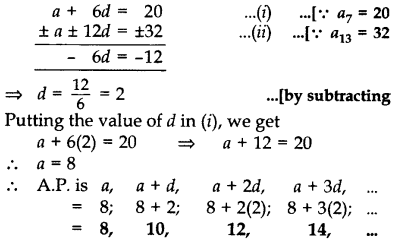Questions On Arithmetic Progression Class 10 Question 14.
Find 10th term from end of the A.P. 4,9, 14, …, 254. (2011OD)
Solution:
Common difference d = 9 – 4
= 14 – 9 = 5
Given: Last term, l = 254, n = 10
nth term from the end = l – (n – 1) d
∴ 10th term from the end = 254 – (10 – 1) × 5
= 254 – 45 = 209

Class 10 Ap Important Questions Question 15.
Find how many two-digit numbers are divisible by 6? (2011OD)
Solution:
12, 18, 24, …,96
Here, a = 12, d = 18 – 12 = 6, an = 96
a + (n – 1)d = an
∴ 12 + (n – 1)6 = 96
⇒ (n − 1)6 = 96 – 12 = 84
⇒ n – 1 = $$\frac{84}{6}$$ = 14
⇒ n = 14 + 1 = 15
∴ There are 15 two-digit numbers divisible by 6.

Question 16.
How many natural numbers are there between 200 and 500, which are divisible by 7? (2011OD)
Solution:
203, 210, 217, …, 497
Here a = 203, d = 210 – 203 = 7, an = 497
∴ a + (n – 1) d = an
203 + (n – 1) 7 = 497
(n – 1) 7 = 497 – 203 = 294
n – 1 = $$\frac{294}{7}$$ = 42 ∴ n = 42 + 1 = 43
∴ There are 43 natural nos. between 200 and 500 which are divisible by 7.

Question 17.
How many two-digit numbers are divisible by 3? (2012OD)
Solution:
Two-digit numbers divisible by 3 are:
12, 15, 18, …, 99
Here, a = 12, d = 15 – 12 = 3, an = 99
∴ a + (n – 1)d = an
12 + (n – 1) (3) = 99
(n – 1) (3) = 99 – 12 = 87
n – 1 = $$\frac{87}{3}$$ = 29
∴ n = 29 + 1 = 30
∴ There are 30 such numbers.

Question 18.
How many three-digit natural numbers are divisible by 7? (2013D)
Solution:
“3 digits nos.” are 100, 101, 102, …, 999
3 digits nos. “divisible by 7” are:
105, 112, 119, 126, …, 994
a = 105, d = 7, an = 994, n = ?
As a + (n – 1)d = 994 = an
∴ 105 + (n – 1)7 = 994
(n − 1)7 = 994 – 105
(n – 1) = $$\frac{889}{7}$$ = 127
∴ n = 127 + 1 = 128

Question 19.
Find the number of all three-digit natural numbers which are divisible by 9. (2013OD)
Solution:
To find: Number of terms of A.P., i.e., n.
A.P. = 108 + 117 + 126 + … + 999
1st term, a = 108
Common difference, d = 117 – 108 = 9
an = 999
a + (n – 1)d = an
∴ 108 + (n – 1) 9 = 999
⇒ (n − 1) 9 = 999 – 108 = 891
⇒ (n − 1) = $$\frac{891}{9}$$ = 99
∴ n = 99 + 1 = 100

Question 20.
Find the number of natural numbers between 101 and 999 which are divisible by both 2 and 5. (2014OD)
Solution:
Numbers divisible by both 2 and 5 are 110, 120, 130, …, 990.
Here a = 110, d = 120 – 110 = 10, an = 990
As a + (n – 1)d = an = 990
110 + (n – 1)(10) = 990
(n – 1)(10) = 990 – 110 = 880
(n − 1) = $$\frac{294}{7}$$ = 88
∴ n = 88 + 1 = 89

Question 21.
Find the middle term of the A.P. 6, 13, 20, …, 216. (2015D)
Solution:
The given A.P. is 6, 13, 20, …, 216
Let n be the number of terms, d = 7, a = 6, an = 216
an = a + (n – 1)d
∴ 216 = 6 + (n – 1).7
216 – 6 = (n – 1)7
$$\frac{210}{7}$$ = n – 1 ⇒ 30 + 1 = n
⇒ n = 31
Middle term = $$\left(\frac{n+1}{2}\right)^{t h}$$ term
= $$\left(\frac{31+1}{2}\right)=\left(\frac{32}{2}\right)$$ = 16th term of the A.P.
∴ a16 = a + 15d = 6 + 15 × 7 = 111

Question 22.
Find the middle term of the A.P. 213, 205, 197, … 37. (2015D)
Solution:
A.P. : 213, 205, 197, …………. 37.
Let a and d be the first term and common difference of A.P. respectively,
Here a = 213, d = -8, an = 37, where n is the number of terms.
an = a + (n – 1)d
∴ 37 = 213 + (n – 1) (-8)
$$\frac{-176}{-8}$$ = n – 1 ⇒ n = 23
∴ Middle term = $$\left(\frac{n+1}{2}\right)^{\text { th }}=\left(\frac{23+1}{2}\right)$$
= 12th term
∴ a12 = a + 11(d) = 213 + 11(-8) = 125

Question 23.
How many terms of the A.P. 27, 24, 21, … should be taken so that their sum is zero? (2016D)
Solution:
Here, 1st term, a = 27
Common difference, d = 24 – 27 = -3
Given: Sn = 0
⇒ $$\frac{n}{2}$$[2a + (n − 1)d] = 0
⇒ $$\frac{n}{2}$$[2(27) + (n − 1)(-3)] = 0
⇒ n(54 – 3n + 3) = 0
⇒ n(57 – 3n) = 0
⇒ n = 0 or (57 – 3n) = 0
⇒ -3n = -57
⇒ n = 19
Since n, i.e., number of terms cannot be zero.
∴ Number of terms = 19

Question 24.
How many terms of the A.P. 65, 60, 55, … be taken so that their sum is zero? (2016D)
Solution:
1st term, a = 65
Common difference, d = 60 – 65 = -5
Sn = 0 …[Given
⇒ $$\frac{n}{2}$$[2a + (n − 1)d] = 0
⇒ $$\frac{n}{2}$$[2(65) + (n − 1)(-5)] = 0
⇒ n(130 – 5n + 5) = 0
⇒ n(135 – 5n) = 0
⇒ n = 0 or 135 – 5n = 0
-5n = -135
⇒ n = 27
Since n, i.e., number of terms can not be zero.
∴ Number of terms = 27

Question 25.
Find the sum of the first 25 terms of an A.P. whose nth term is given by tn = 2 – 3n. (2012D)
Solution:
Given: tn = 2 – 3n
When n = 1, t1 = 2 – 3(1) = -1 ..(i)
When n = 25, t25 = 2 – 3(25) = -73 …(ii)
As Sn = $$\frac{n}{2}$$[t1 + tn], n = 25
∴ S25 = $$\frac{25}{2}$$[-1 + (-73)] .. [From (1) and (ii)
= $$\frac{25 \times(-74)}{2}$$ = 25 × (-37) = -925

Question 26.
The first and the last terms of an AP are 5 and 45 respectively. If the sum of all its terms is 400, find its common difference. (2014D)
Solution:
Here, an = 45, Sn = 400, a = 5, n = ?, d = ?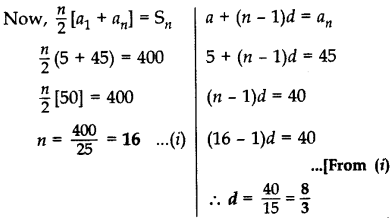Question 27.
The first and the last terms of an AP are 8 and 65 respectively. If the sum of all its terms is 730, find its common difference. (2014D)
Solution:
Here a, = a = 8; an = 65
Given: Sn = 730
⇒ $$\frac{n}{2}$$(8 + 65) = 730 …..[Sn = $$\frac{n}{2}$$(a1 + an)
$$\frac{n}{2}$$(73) = 730
n = 730 × $$\frac{2}{73}$$ = 20
Now, an = a + (n − 1)d = 65
8 +(20 – 1)d = 65 ⇒ 19d = 65 – 8 = 57
∴ d = 3

Question 28.
In an AP, if S5 + S7 = 167 and S10 = 235, then find the AP, where s, denotes the sum of its first n terms. (2015OD)
Solution:
Given: S5 + S7 = 167
⇒ $$\frac{5}{2}$$[2a + (5 – 1)d] + $$\frac{7}{2}$$ [2a + (7 – 1)d] = 167 … [S<sub<n = $$\frac{n}{2}$$ (2a + (n – 1)d)
⇒ $$\frac{5}{2}$$[2a + 4d] + $$\frac{7}{2}$$[2a + 6d] = 167
⇒ 5(a + 2d) + 7(a + 3d) = 167
⇒ 5a + 10d + 7a + 210 = 167
⇒ 12a + 31d = 167
Now, S10 = $$\frac{10}{2}$$ (2a + (10 – 1)d) = 235
⇒ 5[2a + 9d] = 235
⇒ 10a + 45d = 235
Solving (i) and (ii), we get a = 1 and d = 5
a1 = 1
a2 = a + d ⇒ 1 + 5 = 6
a3 = a + 2d ⇒ 1 + 10 = 11
Hence A.P. is 1, 6, 11…

Question 29.
Find the sum of all three digit natural numbers, which are multiples of 11. (2012D)
Solution:
To find: 110 + 121 + 132 + … + 990
Here a = 110, d = 121- 110 = 11, an = 990
∴ a + (n – 1)d = 990
110 + (n – 1).11 = 990
(n – 1). 11 = 990 – 110 = 880
(n – 1) = 880 = 80
n = 80 + 1 = 81
As Sn = $$\frac{n}{2}$$ (a1 + an)
∴ S81 = $$\frac{81}{2}$$ (110 + 990)
= $$\frac{81}{2}$$ (1100) = 81 × 550 = 44,550

### Arithmetic Progressions Class 10 Important Questions Short Answer – II (3 Marks)

Question 30.
Which term of the A.P. 3, 14, 25, 36, … will be 99 more than its 25th term? (2011OD)
Solution:
Let the required term be nth term, i.e., an
Here, d = 14 – 3 = 11, a = 3
According to the Question, an = 99 + a25
∴ a + (n – 1) d = 99 + a + 24d
⇒ (n – 1) (11) = 99 + 24 (11)
(n – 1) (11) = 11 (9 + 24)
n – 1 = 33
n = 33 + 1 = 34
∴ 34th term is 99 more than its 25th term.

Question 31.
Determine the A.P. whose fourth term is 18 and the difference of the ninth term from the fifteenth term is 30. (2011D)
Solution: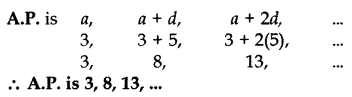Question 32.
The 19th term of an AP is equal to three times its 6th term. If its 9th term is 19, find the A.P. (2013OD)
Solution:
Given: a19 = 3(a6)
⇒ a + 18d = 3(a + 5d)
a + 18d = 3a + 15d
18d – 15d = 3a – a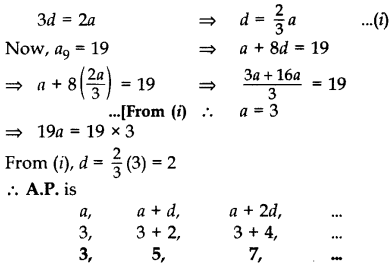Question 33.
The 9th term of an A.P. is equal to 6 times its second term. If its 5th term is 22, find the A.P. (2013OD)
Solution:
9th term = 6 (2nd term)
∴ a +8d = 6 (a + d) …[As an = a + (n – 1)d
a + 8d = 6a + 6d
8d – 6d = 6a – a
2d = 5a
⇒ d = $$\frac{5 a}{2}$$ …(i)
Now, a5 = 22
a + 4d = 22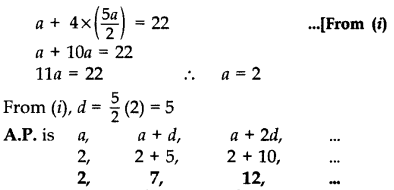Question 34.
The sum of the 5th and the 9th terms of an AP is 30. If its 25th term is three times its 8th term, find the AP. (2014OD)
Solution:
a5 + a9 = 30 … [Given
a + 4d + a + 8d = 30 …[∵ an = a + (n – 1)d
2a + 12d = 30 ⇒ a + 6d = 15 …[Dividing by 2
a = 15 – 6d …(i)
Now, a52 = 3(a8)
a + 24d = 3(a + 7d)
15 – 6d + 240 = 3(15 – 6d + 7d) …[From (i)
15 + 18d = 3(15 + d)
15 + 18d = 45 + 3d
18d – 3d = 45 – 15
15d = 30 ∴ d = $$\frac{30}{15}$$ = 2
From (i), a = 15 – 6(2) = 15 – 12 = 3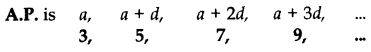Question 35.
If the seventh term of an AP is $$\frac{1}{9}$$ and its ninth term is $$\frac{1}{7}$$, find its 63rd term. (2014D)
Solution:Question 36.
Find the value of the middle term of the following A.P.: -6, -2, 2, …, 58. (2011D)
Solution:
Here a = -6, d = -2 -(-6) = 4, an = 58
As we know, a + (n – 1) d = 58
∴ -6 + (n – 1) 4 = 58
⇒ (n – 1) 4 = 58 + 6 = 64
⇒ (n – 1) = $$\frac{64}{4}$$ = 16
⇒ n = 16 + 1 = 17 (odd)
Middle term = $$\left(\frac{n+1}{2}\right)^{t h}$$ term
$$\left(\frac{17+1}{2}\right)^{t h}$$ term = 9th term
∴ a9 = a + 8d = -6 + 8 (4) = -6 + 32 = 26
∴ Middle term = 26

Question 37.
Find the number of terms of the AP 18, 151/2, 13,…, -491/2 and find the sum of all its terms.
Solution:
Here 1st term, a = 18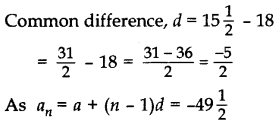Question 38.
The 14th term of an AP is twice its gth term. If its 6th term is -8, then find the sum of its first 20 terms. (2015OD)
Solution:
Let a = First term, d = Common difference
a14 = 2.a8 … [Given
⇒ a + 13d = 2 (a + 7d) ..[∵ a, = a + (n – 1)d
⇒ a + 13d = 2a + 14d
⇒ 1a – 2a = 14d – 13d
⇒ -1a = d ⇒ a = -d … (i)
a6 = -8 …[Given
⇒ -8 = a + 5d
⇒ -d + 5d = -8 …[From (i)
⇒ 4d = -8 ⇒ d = -2
Value of d put in equation (i), we get
a = -d ⇒ a=-(-2)
Now, a = 2, d = -2
Now, Sum of first 20 terms,
S20 = $$\frac{20}{2}$$[2 × 2 + (20 – 1)(-2)] …[Sn = $$\frac{n}{2}$$ (2a + (n – 1)d)
S20 = 10[4 + 19(-2)]
S20 = 10[4 – 38] = -340

Question 39.
The 13th term of an AP is four times its 3rd term. If its fifth term is 16, then find the sum of its first ten terms. (2015OD)
Solution:
a13 = 4a3 … [Given
⇒ a + 12d = 4(a + 2d) …[∵ an = a + (n – 1)d
⇒ a + 12d – 4a – 8d = 0
⇒ 4d = 3a ⇒ d = $$\frac{3 a}{4}$$ …(i)
a5 = 16 … [Given
⇒ a + 4d = 16
⇒ a +4($$\frac{3 a}{4}$$) = 16 … [From (i)
⇒ a + 3a = 16
⇒ 4a = 16 ⇒ a = $$\frac{16}{4}$$ = 4 ..(ii)
Putting a = 4 in (i), we get a = 3
⇒ d = $$\frac{3 \times 4}{4}$$ = 3 … [From (ii)
∴ Sn = $$\frac{n}{2}$$ [2a + (n – 1)d]
S10 = $$\frac{10}{2}$$ [2(4) + (10 – 1)(3)] …[n = 10 (Given)
S10 = 5 (8 + 27) ⇒ 5(35) = 175

Question 40.
If the sum of first 7 terms of an A.P is 49 and that of its first 17 terms is 289, find the sum of first n terms of the A.P. (2016D)
Solution:
Let 1st term = a, Common difference = d
Given: S7 = 49, S17 = 289Question 41.
The first term of an A.P. is 5, the last term is 45 and the sum of all its terms is 400. Find the number of terms and the common difference of the A.P. (2017OD)
Solution:
First term, a = 5, Last term, an = 45
Let the number of terms = n
Sn = 400
$$\frac{n}{2}$$ (a + an) = 400
$$\frac{n}{2}$$(5 + 45) = 400
$$\frac{n}{2}$$ (50) = 400
n = $$\frac{400}{25}$$ = 16 = Number of terms
Now, an = 45
a + (n – 1)d = an
5+ (16 – 1)d = 45
15d = 45 – 5 ∴ d = $$\frac{40}{15}=\frac{8}{3}$$

Question 42.
The nth term of an A.P. is given by (-4n + 15). Find the sum of first 20 terms of this A.P. (2013D)
Solution:
We have, an = -4n + 15
Put n = 1, a1 = -4(1) + 15 = 11
Put n=2, a2 = -4(2) + 15 = 7
∴ d = a2 – a1 = 7 – 11 = -4
As Sn = $$\frac{n}{2}$$ (2a + (n – 1)d]
∴ S20 = $$\frac{20}{2}$$ [2(11) + (20 – 1)(-4))… [n = 20 (Given)
= 10 (22 – 76)
= 10 (-54) = -540

Question 43.
The sum of first n terms of an AP is 3n2 + 4n. Find the 25th term of this AP. (2013D)
Solution:
We have, Sn = 3n2 + 4n
Put n = 25,
S25 = 3(25)2 + 4(25)
= 3(625) + 100
= 1875 + 100 = 1975
Put n = 24,
S24 = 3(24)2 + 4(24)
= 3(576) + 96
= 1728 + 96 = 1824
∴ 25th term = S25 – S24
= 1975 – 1824 = 151

Question 44.
The sum of the first seven terms of an AP is 182. If its 4th and the 17th terms are in the ratio 1 : 5, find the AP. (2014OD)
Solution:
S7 = 182 …[Given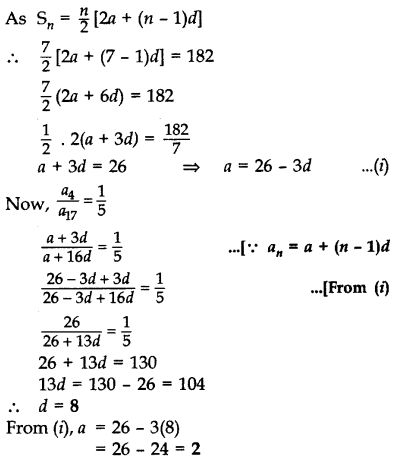∴ a = 2, d = 8
∴AP is 2, 10, 18, 26, 34, …

Question 45.
If Sn, denotes the sum of first n terms of an A.P., prove that S12 = 3(S8 – S4). (2015D)
Solution:
Let a be the first term and d be the common difference of A.P.
Sn = $$\frac{n}{2}$$ (2a + (n − 1)d)
∴ S12 = $$\frac{12}{2}$$ (2a + (12 – 1)d)
S12 = 6 [2a + 11d] = 124 + 66d …(i)
∴ S8 = $$\frac{8n}{2}$$ (2a + (8 – 1)d)
S8 = 4[2a + 7d] = 8a + 28d … (ii)
∴ S4 = $$\frac{4}{2}$$ (2a + (4 – 1)d)
S4 = 2[2a + 3d) = 4a + 6d …(iii)
Now, S12 = 3(S8 – S4)
12a + 660 = 3(8a + 28d – 4a – 6d) … [From (i), (ii) & (iii)
12a + 660 = 3(4a + 22d)
12a + 660 = 12a + 66d …Hence proved

Question 46.
If the sum of the first n terms of an A.P. is $$\frac{1}{2}$$ (3n2 + 7n), then find its nth term. Hence write its 20th (2015D)
Solution:
Sn = $$\frac{1}{2}$$ (3n2 + 7n) …[Given
Put n = 1
S1 = $$\frac{1}{2}$$[3(1)2 + 7(1)] = $$\frac{1}{2}$$ [3 + 7) = $$\frac{1}{2}$$ (10) = 5
Put n = 2
S2 = $$\frac{1}{2}$$ [3(2)2 + 7(2)] = $$\frac{1}{2}$$[3(4) + 7(2)]
= S2 = $$\frac{1}{2}$$(12 + 14) = $$\frac{1}{2}$$ (26) = 13
Now we know,
S1 = 27 and a2 = S2 – S1
∴ a1 = 5 = 13 – 5 = 8
Now we have,
a1 = 5, a2 = 8, d = a2 – a1 = 8 – 5 = 3
an = a + (n – 1)d = 5 + (n – 1)3
= 5 + 3n – 3 = 3n + 2 (nth term)
∴ 20th term, a20 = (3 × 20) + 2 = 62

Question 47.
If Sn denotes the sum of first n terms of an A.P., prove that S30 = 3[S20 – S10]. (2015D)
Solution:
Let a be the first term and d be the common difference of the A.P.
Sn = $$\frac{n}{2}$$[2a + (n − 1)d]
S30 = $$\frac{30}{2}$$ [2a + (30 – 1)d] = 15[2a + 29d]
= 30a + 435d …(i)
S20 = $$\frac{20}{2}$$ [2a + (20 – 1)d] = 10[2a + 19d]
= 20a + 190d …(ii)
S10 = $$\frac{10}{2}$$[2a + (10 – 1)d] = 5[2a + 9d]
= 10a + 45d …(iii)
To prove, 3(S20 – S10) = S30
= 3(20a + 190d – 10a – 45d) …[From (i), (ii) & (iii)
= 3(10a + 145d)
= 30a + 435d = S30 …Hence Proved

Question 48.
If the ratio of the sum of first n terms of two A.Ps is (7n + 1): (4n + 27), find the ratio of their mth terms. (2016OD)
Solution:
Let A be first term and D be the common difference of 1st A.P.
Let a be the first term and d be the common difference of 2nd A.P.∴ Required ratio = (14m – 6) : (8m + 23)

Question 49.
The digits of a positive number of three digits are in A.P. and their sum is 15. The number obtained by reversing the digits is 594 less than the original number. Find the number. (2016OD)
Solution:
Let hundred’s place digit = (a – d)
Let ten’s place digit = a
Let unit’s place digit = a + d
According to the Question,
a – d + a + a + d = 15
⇒ 3a = 15 ⇒ a = 5
Original number
= 100(a – d) + 10(a) + 1(a + d)
= 100a – 100d + 10a + a + d
= 111a – 99d
Reversed number
= 1(a – d) + 10a + 100(a + d)
= a – d + 10a + 100a + 100d
= 111a + 99d
Now, Original no. – Reversed no. = 594
111a – 99d – (111a + 99d) = 594
-198d = 594 ⇒ d = $$\frac{594}{-198}$$ = -3
∴ The Original no. = 111a – 99d
= 111(5) – 99(-3)
= 555 + 297 = 852

Question 50.
The sums of first n terms of three arithmetic progressions are S1 S2 and S3 respectively. The first term of each A.P. is 1 and their common differences are 1, 2 and 3 respectively. Prove that S1 + S3 = 2S2. (2016OD)
Solution: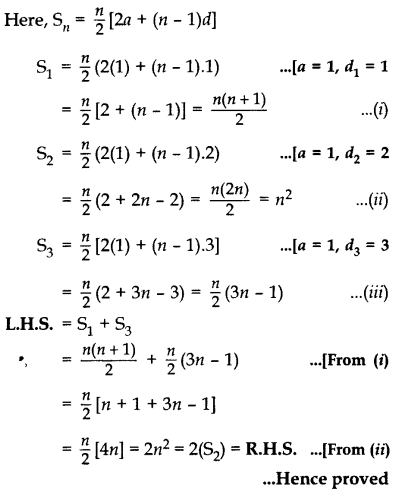Question 51.
Find the sum of all three digit natural numbers, which are multiples of 9. (2012D)
Solution:
To find: 108 + 117 + 126 + … + 999
1st term, a = 108
Common difference, d = 117 – 108 = 9
∴ a + (n – 1)d = an = 999
108 + (n – 1). 9 = 999
(n – 1)9 = 999 – 108 = 891
(n – 1) = $$\frac{891}{9}$$ = 99
n = 99 + 1 = 100
As Sn= $$\frac{n}{2}$$(a1 + an)
∴ S100 = $$$\frac{100}{2}$$$ (108 + 999)
= 50(1107) = 55350

Question 52.
Find the sum of all multiples of 7 lying between 500 and 900. (2012OD)
Solution:
To find: 504 + 511 + 518 + … + 896
a = 504, d = 511- 504 = 7, an = 896
a + (n – 1)d = an
∴ 504 + (n – 1)7 = 896
(n – 1)7 = 896 – 504 = 392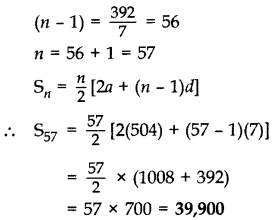### Arithmetic Progressions Class 10 Important Questions Long Answer (4 Marks)

Question 53.
If pth, qth and rth terms of an A.P. are a, b, c respectively, then show that (a – b)r + (b – c)p+ (c – a)q = 0. (2011D)
Solution:
Let A be the first term and D be the common difference of the given A.P.
pth term = A + (p – 1)D = a …(i)
qth term = A + (q – 1)D = b …(ii)
rth term = A + (r – 1)D = c … (iii)
L.H.S. = (a – b)r + (b – c)p + (c – a)q
= [A + (p – 1)D – (A + (q – 1)D)]r + [A + (q – 1)D – (A + (r – 1)D)]p + [A + (r – 1)D – (A + (p – 1)D)]q
= [(p – 1 – q + 1)D]r + [(q – 1 – r + 1)D]p + [(r – 1 – p + 1)D]q
= D[(p – q)r + (q – r)p + (r – p)q]
= D[pr – qr + qp – rp + rq – pq]
= D = 0 = R.H.S.

Question 54.
The 17th term of an AP is 5 more than twice its 8th term. If the 11th term of the AP is 43, then find its nth term. (2012D)
Solution: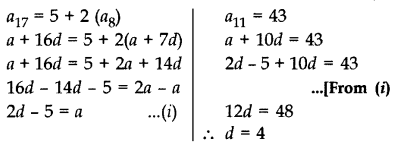From (i),
a = 2(4) – 5 = 8 – 5 = 3
As an = a + (n – 1) d
∴ an = 3 + (n – 1) 4 = 3 + 4n – 4
an = (4n – 1)

Question 55.
The 15th term of an AP is 3 more than twice its 7th term. If the 10th term of the AP is 41, then find its nth term. (2012D)
Solution:From (i),
a = 2(4) – 3
= 8 – 3 = 5
nth term = a + (n – 1) d
∴ nth term = 5 + (n – 1) 4
= 5 + 4n – 4 = (4n + 1)

Question 56.
The 16th term of an AP is 1 more than twice its 8th term. If the 12th term of the AP is 47, then find its nth term. (2012D)
Solution:From (i) and (ii), a = 4 – 1 = 3
As nth term = a + (n – 1) d
∴ nth term = 3 + (n – 1) 4
= 3 + 4n -4 = 4n -1

Question 57.
A sum of ₹1,600 is to be used to give ten cash prizes to students of a school for their overall academic performance. If each prize is ₹20 less than its preceding prize, find the value of each of the prizes. (2012OD)
Solution:
Here S10 = 1600, d = -20, n = 10
Sn = $$\frac{n}{2}$$ (2a + (n – 1)d]
∴ $$\frac{10}{2}$$[2a + (10 – 1)(-20)] = 1600
2a – 180 = 320
2a = 320 + 180 = 500
a = 250
∴ 1st prize = a = ₹250
2nd prize = a2 = a + d = 250 + (-20) = ₹230
3rd prize = a3 = a2 + d = 230 – 20 = ₹210
4th prize = a4 = a3 + d = 210 – 20 = ₹190
5th prize = a5 = a4 + d = 190 – 20 = ₹170
6th prize = a6 = a5 + d = 170 – 20 = ₹150
7th prize = a7 = a6 + d = 150 – 20 = ₹130
8th prize = a8 = a7 + d = 130 – 20 = ₹110
9th prize = a9 = a8 + d = 110 – 20 = ₹590
10th prize = a10 = a9 + d = 90 – 20 = ₹70
= ₹ 1,600

Question 58.
Find the 60th term of the AP 8, 10, 12, …, if it has a total of 60 terms and hence find the sum of its last 10 terms. (2015OD)
Solution:
a = 8, d = a2 – a1 = 10 – 8 = 2, n = 60
a60 = a + 59d = 8 + 59(2) = 126
∴ Sum of its last 10 terms = S60 – S50
= $$\frac{n}{2}$$(a + an) – $$\frac{n}{2}$$(2a + (n – 1)d)
= $$\frac{60}{2}$$ (8 + a60) – $$\frac{50}{2}$$ (2 × 8 + (50 – 1)2)
= 30 (8 + 126) – 25 (16 + 98)
= 4020 – 25 × 114
= 4020 – 2850 = 1170

Question 59.
An Arithmetic Progression 5, 12, 19, … has 50 terms. Find its last term. Hence find the sum of its last 15 terms. (2015OD)
Solution:
Let a and d be the first term and common difference of A.P. respectively,
a = 5, d = 12 – 5 = 7, n = 50
∴ an = a + (n – 1)d
a50 = 5 + 49(7) = 5 + 343 = 348
∴ Sum of its last 15 terms = S50 – S35
= $$\frac{n}{2}$$(a + an) – $$\frac{n}{2}$$ (2a + (n − 1)d)
= $$\frac{50}{2}$$ (5 + 348) – $$\frac{35}{2}$$ [2(5) + (35 – 1)7]
= 25(353) – $$\frac{35}{2}$$ (10 + 238)
= 8825 – 35 × 124
= 8825 – 4340 = 4485

Question 60.
If the sum of first 4 terms of an A.P. is 40 and that of first 14 terms is 280, find the sum of its first n terms. (2011D)
Solution:
Sn = $$\frac{n}{2}$$[2a + (n − 1) d] …(i)Putting the value of d = 2 in (ii), we get a = 7
∴ Sn = $$\frac{n}{2}$$[2(7) + (n – 1). 2]
= $$\frac{n}{2}$$.2 [7 + n – 1]
= n (n + 6) or n2 + 6n

Question 61.
The first and the last terms of an A.P. are 8 and 350 respectively. If its common difference is 9, how many terms are there and what is their sum? (2011D)
Solution:
Here a = 8, an = 350, d = 9
As we know, a + (n − 1) d = a2
∴ 8 + (n – 1) 9 = 350
(n − 1) 9 = 350 – 8 = 342
n – 1 = $$\frac{342}{9}$$ = 38
n = 38 + 1 = 39
∴ There are 39 terms.
∴ Sn = $$\frac{n}{2}$$(a + an)
∴ S39 = $$\frac{39}{2}$$ (8 + 350) = $$\frac{39}{2}$$ × 358
= 39 × 179 = 6981

Question 62.
Sum of the first 20 terms of an AP is -240, and its first term is 7. Find its 24th term. (2012D)
Solution:
Given: a = 7, S20 = -240
Here, Sn = $$\frac{n}{2}$$[2a + (n – 1)d]
∴ S20 = $$\frac{20}{2}$$[2(7) + (20 – 1)d]
-240 = 10(14 + 19d)
–$$\frac{240}{10}$$ = 14 + 19d = -24
19d = -24 – 14 = -38
⇒ d = $$\frac{-38}{19}$$ = -2 …(i)
Again, an = a + (n – 1)d
∴ a24 = 7 + (24 – 1) (-2) … [From (i)
= 7 – 46 = -39

Question 63.
Find the common difference of an A.P. whose first term is 5 and the sum of its first four terms is half the sum of the next four terms. (2012OD)
Solution:
Here a = 5 … (i)
Here, a1 + a2 + a3 + a4 = $$\frac{1}{2}$$ (a5 + a6 + a7 + a8)
∴ a + (a + d) + (a + 2d) + (a + 3d)
= $$\frac{1}{2}$$ [(a + 4d) + (a + 5d) + (a + 6d) + (a + 780] …[an = a(n – 1)d
∴ 4a + 6d = $$\frac{1}{2}$$ (4a + 22d)
8a + 12d = 4a + 22d
8a – 4a = 22d – 12d
4a = 100 ⇒ 4(5) = 10d
d = $$\frac{4(5)}{10}=\frac{20}{10}$$ = 2 …[From (1)
∴ Common difference, d = 2

Question 64.
If the sum of the first 7 terms of an A.P. is 119 and that of the first 17 terms is 714, find the sum of its first n terms. (2012OD)
Solution:
As Sn = $$\frac{n}{2}$$[2a + (n − 1)d]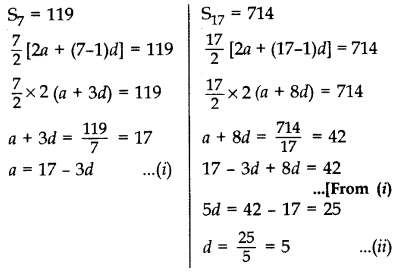S2 = 119
From (i) and (ii), a = 17 – 3(5) = 17 – 15 = 2
∴Sn = $$\frac{1}{2}$$[2(2) + (n – 1)5]
= $$\frac{n}{2}$$[4 + 5n – 5]
= $$\frac{n}{2}$$ (5n – 1)

Question 65.
The 24th term of an AP is twice its 10th term. Show that its 72nd term is 4 times its 15th term. (2013D)
Solution:
a24 = 2 (10) … [Given
a + 23d = 2 (a +9d) [∵ an = a + (n – 1)d)
23d = 2a + 18d – a
23d – 18d = a ⇒ a = 5d …(i)
To prove: a72 = 4 (a15)From (ii) and (iii), L.H.S = R.H.S …Hence Proved

Question 66.
Find the number of terms of the A.P. -12, -9, – 6, …, 21. If 1 is added to each term of this A.P., then find the sum of all terms of the A.P. thus obtained. (2013D)
Solution:
Here a = -12, d=-9 + 12 = 3
Here, an = a + (n – 1)d = 21
∴ -12 + (n – 1).3 = 21
(n – 1).3 = 21 + 12 = 33
∴ n – 1 = 11
Total number of terms,
n = 11 + 1 = 12
New A.P. isHere 1st term, a = -11
Common difference, d = -8 + 11 = 3;
Last term, an = 22; Number of terms, n= 12Question 67.
In an AP of 50 terms, the sum of first 10 terms is 210 and the sum of its last 15 terms is 2565. Find the AP. (2014D)
Solution:
Here, n = 50,
Here, S10 = 210
= $$\frac{10}{2}$$ (2a + 9d) = 210 ….[Sn = $$\frac{1}{2}$$ [2a+(n – 1)2]
5(2a + 9d) = 210
2a + 9d = $$\frac{210}{5}$$ = 42
⇒ 2a = 42 – 9d ⇒ a = $$\frac{42-9 d}{2}$$ …(i)
Now, 50 = (1 + 2 + 3 + …) + (36 + 37 + … + 50) Sum = 2565
Sum of its last 15 terms = 2565 …[Given
S50 – S35 = 2565
$$\frac{50}{2}$$(2a + 49d) – $$\frac{35}{2}$$ (2a + 34d) = 2565
100a + 2450d – 70a – 1190d = 2565 × 2
30a + 1260d = 5130
3a + 1260 = 513 …[Dividing both sides by 10Question 68.
If the ratio of the sum of the first n terms of two A.Ps is (7n + 1): (4n + 27), then find the ratio of their 9th terms. (2017OD)
Solution: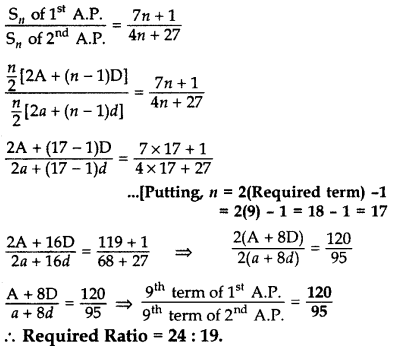Question 69.
In a school, students decided to plant trees in and around the school to reduce air pollution. It was decided that the number of trees, that each section of each class will plant, will be double of the class in which they are studying. If there are 1 to 12 classes in the school and each class has two Sections, find how many trees were planted by the students. (2014OD)
Solution:
Classes: 1 + I + II + … + XII
Sections: 2(I) + 2(II) + 2(III) + … + 2(XII)
Total no. of trees
= 2 + 4 + 6 … + 24
= (2 × 2) + (2 × 4) + (2 × 6) + … + (2 × 24)
= 4 + 8 + 12 + … + 48
:: S12 = $$\frac{12}{2}$$(4 + 48) = 6(52) = 312 trees

Question 70.
Ramkali required ₹500 after 12 weeks to send her daughter to school. She saved ₹100 in the first week and increased her weekly saving by ₹20 every week. Find whether she will be able to send her daughter to school after 12 weeks. (2015D)
Solution:
Money required by Ramkali for admission of her daughter = ₹2500
A.P. formed by saving
100, 120, 140, … upto 12 terms ….(i)
Let, a, d and n be the first term, common difference and number of terms respectively. Here, a = 100, d = 20, n = 12
Sn = $$\frac{n}{2}$$ (2a + (n − 1)d)
⇒ S12 = $$\frac{12}{2}$$ (2(100) + (12 – 1)20)
S12 = $$\frac{12}{2}$$ [2(100) + 11(20)] = 6 = ₹2520

Question 71.
The sum of first n terms of an A.P. is 5n2 + 3n. If its mth term is 168, find the value of m. Also find the 20th term of this A.P. (2013D)
Solution: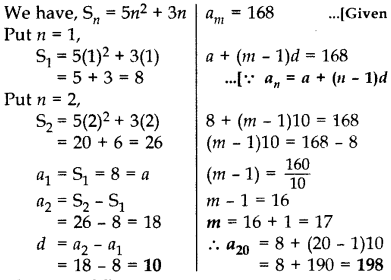Question 72.
The sum of first m terms of an AP is 4m2 – m. If its nth term is 107, find the value of n. Also find the 21st term of this A.P. (2013D)
Solution:
We have, Sm = 4m2 – m
Put m = 1,
S1 = 4(1)2 – (1)
= 4 – 1 = 3
Put m = 2,
S2 = 4(2)2 – 2
= 16 – 2 = 14
∴ a1 = S1 = 3 = a
a2 = S2 – S1 = 14 – 3 = 11
d = a2 – a1
d = 11 – 3 = 8
an = a + (n – 1)d = 107 …[Given
∴ 3+ (n – 1)8 = 107
(n – 1)8 = 107 – 3 = 104
(n – 1) = $$\frac{104}{8}$$ = 13
n = 13 + 1 = 14
∴ a21 = a + 20d
= 3 + (20)8
= 3 + 160 = 163

Question 73.
The sum of first g terms of an A.P. is 63q – 3q2. If its pth term is -60, find the value of p. Also find the 11th term of this A.P. (2013D)
Solution:
We have, Sq = 63q – 3q2
Put q = 1,
S7 = 63(1) – 3(1)2
= 63 – 3 = 60
Put q = 2,
S2 = 63(2) – 3(2)2
= 126 – 12 = 114
∴ a = a1 = S1 = 60
a2 = S2 – S71 = 114 – 60 = 54
d = a2 – a1 = 54 – 60 = -6
pth term = -60
a + (p – 1)d = -60
60 + (p – 1)(-6) = -60
(p – 1)(-6) = -60 – 60 = -120
(p – 1) = $$\frac{-120}{-6}$$ = 20
p= 20 + 1 = 21
∴ a11 = a + 10d
= 60 + 10(-6)
= 60 – 60 = 0

Question 74.
Find the sum of the first 30 positive integers divisible by 6. (2011D)
Solution:
To find: 6 + 12 + 18 + … (30 terms)
Here a = 6, d = 12 – 6 = 6, n = 30
Sn = [2a + (n − 1) d]
∴ S30 = $$\frac{30}{2}$$[2(6) + (30 – 1) 6]
= 15 [12 + 29(6)]
= 15 (12 + 174)
= 15 (186) = 2790

Question 75.
How many multiples of 4 lie between 10 and 250? Also find their sum. (2011D)
Solution:
Multiples of 4 between 10 and 250 are:
12, 16, 20, ……… 248
Here, a = 12, d = 4, an = 248
As we know, a + (n – 1) d = an
∴12 + (n – 1) 4 = 248
⇒ (n – 1) 4 = 248 – 12 = 236
n – 1 = $$\frac{236}{4}$$ = 59
⇒ n = 59 + 1 = 60
∴ There are 60 terms.
Now, Sn = $$\frac{n}{2}$$ (a + an)
∴ S60 = $$$\frac{60}{2}$$$(12 + 248)
= 30 (260) = 7800

Question 76.
Find the sum of all multiples of 8 lying between 201 and 950. (2012OD)
Solution:
To find: 208 + 216 +224 + … + 944
Here, a = 208, d = 216 – 208 = 8, an = 944
a + (n – 1)d = an
∴ 208 + (n – 1)8 = 944
(n – 1)8 = 944 – 208 = 736
n – 1 = $$\frac{736}{8}$$ = 92
8 n = 92 + 1 = 93
Now, Sn = $$\frac{n}{2}$$(a1 + an)
:: S93 = $$\frac{93}{2}$$ (208 + 944)
= $$\frac{93}{2}$$ × 1152 = 93 × 576 = 53568

Question 77.
Find the sum of all multiples of 9 lying between 400 and 800. (2012OD)
Solution:
To find: 405 + 414 + 423 + … +792
Here a = 405, d = 414 – 405 = 9, an = 792
a + (n – 1)d = an
∴ 405 + (n – 1)9 = 792
(n – 1)9 = 792 – 405 = 387
n – 1 = $$\frac{387}{9}$$ = 43
∴ n = 43 + 1 = 44
As Sn = $$\frac{n}{2}$$(a1 + an)
∴ S44 = $$\frac{44}{2}$$ (405 + 792)
= 22 × 1197 = 26334

Question 78.
A thief runs with a uniform speed of 100 m/minute. After one minute a policeman runs after the thief to catch him. He goes with a speed of 100 m/minute in the first minute and increases his speed by 10 m/minute every succeeding minute. After how many minutes the policeman will catch the thief. (2016D)
Solution:
Let total time ben minutes. Total distance covered by thief in n minutes
= Speed × Time
= 100 × n = 100 n metres
Total distance covered by policeman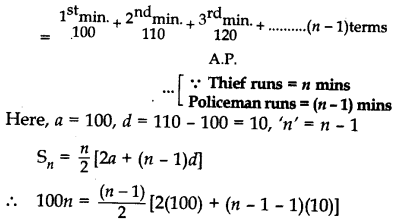⇒ (n – 1) (200 + 10n – 20) = 200n
⇒ (n – 1) [10n + 180) = 200n
⇒ 10n2 + 180n – 10n – 180 – 200n = 0
⇒ 10n2 – 30n – 180 = 0
⇒ n2 – 3n – 18 = 0 … [Dividing both sides by 10
⇒ n2 – 6n + 3n – 18 = 0
⇒ n(n – 6) + 3(n – 6) = 0
⇒ (n + 3) (n – 6) = 0
⇒ n + 3 = 0 or n – 6 = 0
⇒ n = -3 or n = 6
But n (time) can not be negative.
∴ Time taken by policeman to catch the thief
= n – 1 = 6 – 1 = 5 minutes

Question 79.
A thief, after committing a theft, runs at a uniform speed of 50 m/minute. After 2 minutes, a policeman runs to catch him. He goes 60 m in first minute and increases his speed by 5 m/ minute every succeeding minute. After how many minutes, the policeman will catch the thief? (2016D)
Solution:
Let total time be n minutes.
Total distance covered by thief in n minutes
= Speed × Time
= (50 × n) metres = 50 n metres
Total distance covered by policeman⇒ (n – 2) (120 + 5n – 15) = 100n
⇒ (n – 2) [5n + 105) = 100n
⇒ 5n2 + 105n – 10n – 210 – 100n = 0
⇒ 5n2 – 5n – 210 = 0
⇒ n2 – n – 42 = 0 …[Dividing both sides by 5
⇒ n2 – 7n + 6n – 42 = 0
⇒ n(n – 7) + 6(n – 7) = 0
⇒ (n – 7) (n + 6) = 0
⇒ n – 7 = 0 or n + 6 = 0
⇒ n = 7 or n = -6 (reject)
But n (time) can not be negative.
∴ Time taken by policeman to catch the thief
= n – 2 = 7 – 2 = 5 minutes

Question 80.
The houses in a row are numbered conse cutively from 1 to 49. Show that there exists a value of X such that sum of numbers of houses preceding the house numbered X is equal to sum of the numbers of houses following X. (2016OD)
Solution:
Here the A.P. is 1, 2, 3, …., 49
Here a = 1, d = 1, an = 49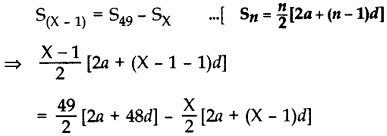Now,
⇒ (X – 1)2 (2 + (X – 2)) = 49(2 + 48) – X[2 + (x – 1)]
⇒ (X – 1). X = 2,450 – X(X + 1)
⇒ x2 – X = 2,450 – X2 – X
⇒ X2 – X + X2 + X = 2,450
⇒ 2X2 = 2,450
⇒ X2 = 1,225
∴ X = $$+\sqrt{1,225}$$ = 35 …[X can not be -ve

Question 81.
Find the sum of n terms of the series $$\left(4-\frac{1}{n}\right)+\left(4-\frac{2}{n}\right)+\left(4-\frac{3}{n}\right)+\ldots \ldots .$$ (2017D)
Solution:
First term, a = 4 – $$\frac{1}{n}$$